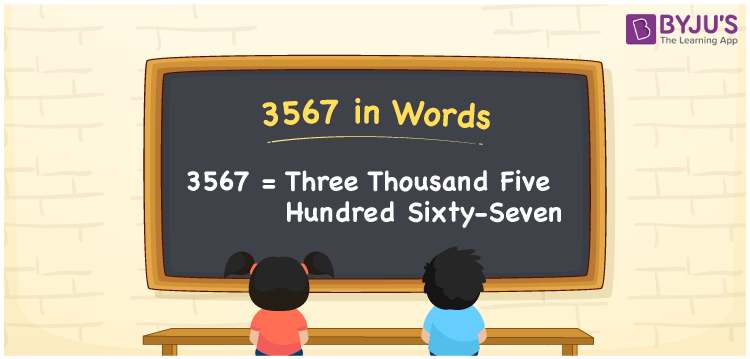# 3567 in Words

3567 in words is expressed as Three Thousand Five Hundred Sixty-Seven. For example, Aasha purchased a silk saree worth Rs. 3567, then you can say, “Aasha purchased a silk saree worth Rupees Three Thousand Five Hundred Sixty-Seven”. The number 3567 is a cardinal number as it denotes the specific amount or value. In this article, let us learn how to write and spell the number 3567 in English along with its conversion into words in detail.

 3567 in Words Three Thousand Five Hundred Sixty-Seven Three Thousand Five Hundred Sixty-Seven in numerical form 3567

## 3567 in English Words

We generally write numbers in words with the help of the English alphabet to help students understand the numbers in an effective way. Therefore, the number 3567 in English can be spelt as Three Thousand Five Hundred Sixty-Seven.## How to Write 3567 in Words?

We can convert the number 3567 quickly using a place value system. The place value chart for the number 3567 is given below.

 Thousands Hundreds Tens Ones 3 5 6 7

Hence, we can write the expanded form as:

3 x Thousand + 5 x 100 + 6 x Ten + 7 x One

= 3 x 1000 + 5 x 100 + 6 x 10 + 7 x 1

= 3000 + 500 + 60 + 7

= 3567

= Three Thousand Five Hundred Sixty-Seven

Therefore, 3567 in words is written as Three Thousand Five Hundred Sixty-Seven

Interesting way of writing 3567 in words

3 = Three

35 = Thirty-Five

356 = Three Hundred and Fifty-Six

3567 = Three Thousand Five Hundred Sixty-Seven

Thus, the word form of the number 3567 is Three Thousand Five Hundred Sixty-Seven

3567 is a natural number that precedes 3568 and succeeds 3566

• 3567 in words – Three Thousand Five Hundred Sixty-Seven
• Is 3567 an odd number? – Yes
• Is 3567 an even number? – No
• Is 3567 a perfect square number? – No
• Is 3567 a perfect cube number? – No
• Is 3567 a prime number? – No
• Is 3567 a composite number? – Yes

## Frequently Asked Questions on 3567 in Words

Q1

### How do you write 3567 in words?

3567 in words is written as Three Thousand Five Hundred Sixty-Seven.
Q2

### Simplify 2000 + 1567, and express in words.

Simplifying 2000 + 1567, we get 3567. Therefore, the number 3567 in words is Three Thousand Five Hundred Sixty-Seven.
Q3

### Convert Three Thousand Five Hundred Sixty-Seven in numbers.

Three Thousand Five Hundred Sixty-Seven in numbers is 3567.